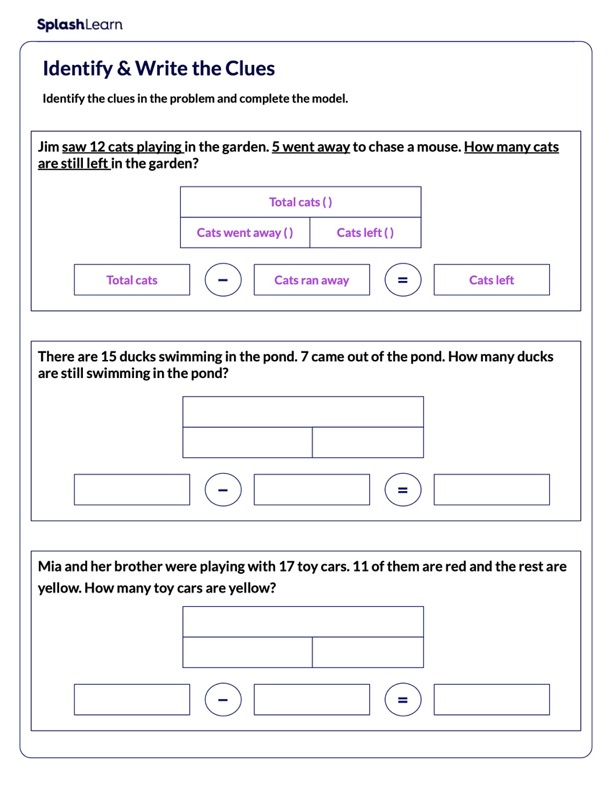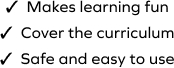# Write Subtraction Equation using Clues

SplashLearn - The complete PreK - Grade 5 Math & ELA Learning Program Built for Your Child
Home > Write Subtraction Equation using CluesBoost your child's problem-solving skills with this worksheet. Students will apply subtraction to real-world situations. They will work with a variety of scenarios. The worksheet requires them to make sense of each story situation and represent the situation using equations. The worksheet involves numbers within 20, it is important for students to gain confidence in a concept by working at different levels of complexity.